# Use rolling-ball algorithm for estimating background intensity#

The rolling-ball algorithm estimates the background intensity of a grayscale image in case of uneven exposure. It is frequently used in biomedical image processing and was first proposed by Stanley R. Sternberg in 1983 .

The algorithm works as a filter and is quite intuitive. We think of the image as a surface that has unit-sized blocks stacked on top of each other in place of each pixel. The number of blocks, and hence surface height, is determined by the intensity of the pixel. To get the intensity of the background at a desired (pixel) position, we imagine submerging a ball under the surface at the desired position. Once it is completely covered by the blocks, the apex of the ball determines the intensity of the background at that position. We can then roll this ball around below the surface to get the background values for the entire image.

Scikit-image implements a general version of this rolling-ball algorithm, which allows you to not just use balls, but arbitrary shapes as kernel and works on n-dimensional ndimages. This allows you to directly filter RGB images or filter image stacks along any (or all) spacial dimensions.

## Classic rolling ball#

In scikit-image, the rolling ball algorithm assumes that your background has low intensity (black), whereas the features have high intensity (white). If this is the case for your image, you can directly use the filter like so:

```import matplotlib.pyplot as plt
import numpy as np
import pywt

from skimage import (
data, restoration, util
)

def plot_result(image, background):
fig, ax = plt.subplots(nrows=1, ncols=3)

ax.imshow(image, cmap='gray')
ax.set_title('Original image')
ax.axis('off')

ax.imshow(background, cmap='gray')
ax.set_title('Background')
ax.axis('off')

ax.imshow(image - background, cmap='gray')
ax.set_title('Result')
ax.axis('off')

fig.tight_layout()

image = data.coins()

background = restoration.rolling_ball(image)

plot_result(image, background)
plt.show()
```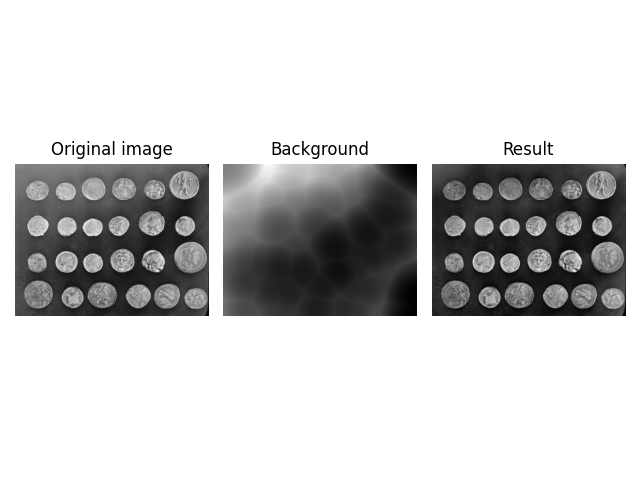## White background#

If you have dark features on a bright background, you need to invert the image before you pass it into the algorithm, and then invert the result. This can be accomplished via:

```image = data.page()
image_inverted = util.invert(image)

filtered_image_inverted = image_inverted - background_inverted
filtered_image = util.invert(filtered_image_inverted)
background = util.invert(background_inverted)

fig, ax = plt.subplots(nrows=1, ncols=3)

ax.imshow(image, cmap='gray')
ax.set_title('Original image')
ax.axis('off')

ax.imshow(background, cmap='gray')
ax.set_title('Background')
ax.axis('off')

ax.imshow(filtered_image, cmap='gray')
ax.set_title('Result')
ax.axis('off')

fig.tight_layout()

plt.show()
```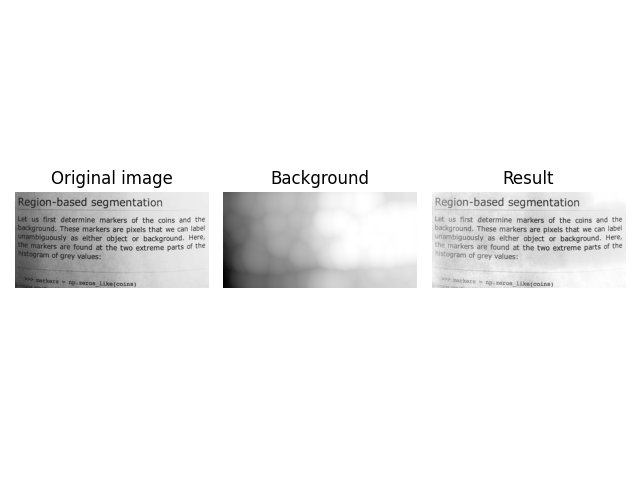Be careful not to fall victim to an integer underflow when subtracting a bright background. For example, this code looks correct, but may suffer from an underflow leading to unwanted artifacts. You can see this in the top right corner of the visualization.

```image = data.page()
image_inverted = util.invert(image)

background = util.invert(background_inverted)
underflow_image = image - background  # integer underflow occurs here

# correct subtraction
correct_image = util.invert(image_inverted - background_inverted)

fig, ax = plt.subplots(nrows=1, ncols=2)

ax.imshow(underflow_image, cmap='gray')
ax.set_title('Background Removal with Underflow')
ax.axis('off')

ax.imshow(correct_image, cmap='gray')
ax.set_title('Correct Background Removal')
ax.axis('off')

fig.tight_layout()

plt.show()
```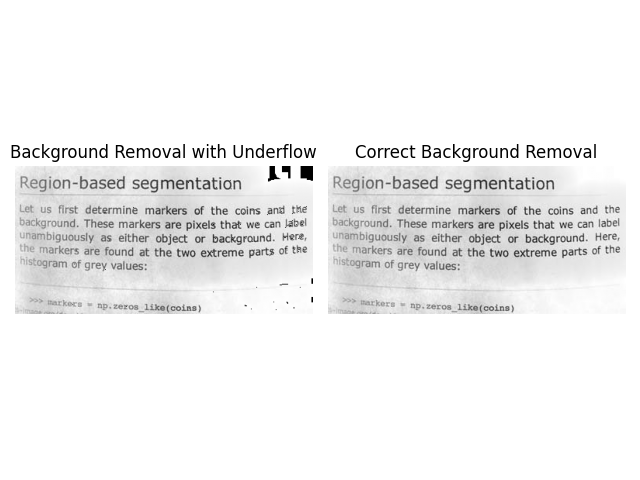## Image Datatypes#

`rolling_ball` can handle datatypes other than np.uint8. You can pass them into the function in the same way.

```image = data.coins()[:200, :200].astype(np.uint16)

plot_result(image, background)
plt.show()
```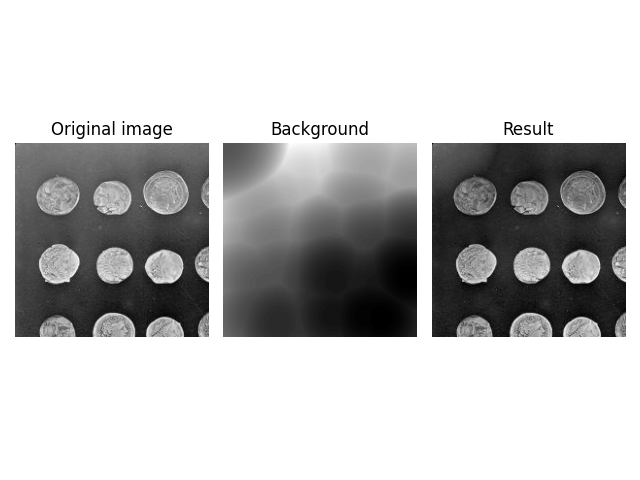However, you need to be careful if you use floating point images that have been normalized to `[0, 1]`. In this case the ball will be much larger than the image intensity, which can lead to unexpected results.

```image = util.img_as_float(data.coins()[:200, :200])

plot_result(image, background)
plt.show()
```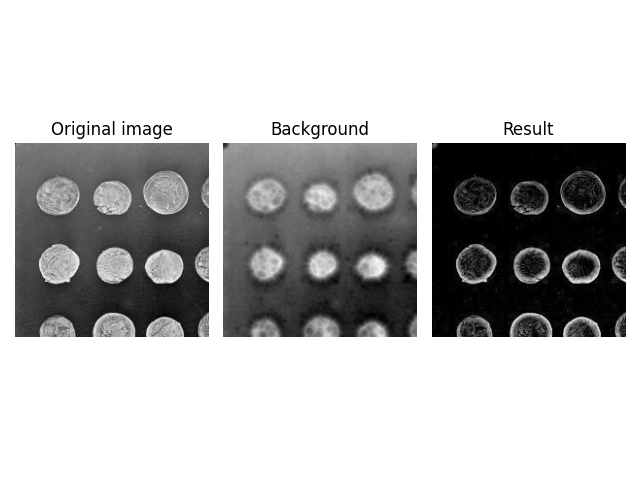Because `radius=70.5` is much larger than the maximum intensity of the image, the effective kernel size is reduced significantly, i.e., only a small cap (approximately `radius=10`) of the ball is rolled around in the image. You can find a reproduction of this strange effect in the `Advanced Shapes` section below.

To get the expected result, you need to reduce the intensity of the kernel. This is done by specifying the kernel manually using the `kernel` argument.

Note: The radius is equal to the length of a semi-axis of an ellipsis, which is half a full axis. Hence, the kernel shape is multiplied by two.

```normalized_radius = 70.5 / 255
image = util.img_as_float(data.coins())
kernel = restoration.ellipsoid_kernel(
(70.5 * 2, 70.5 * 2),
)

background = restoration.rolling_ball(
image,
kernel=kernel
)
plot_result(image, background)
plt.show()
```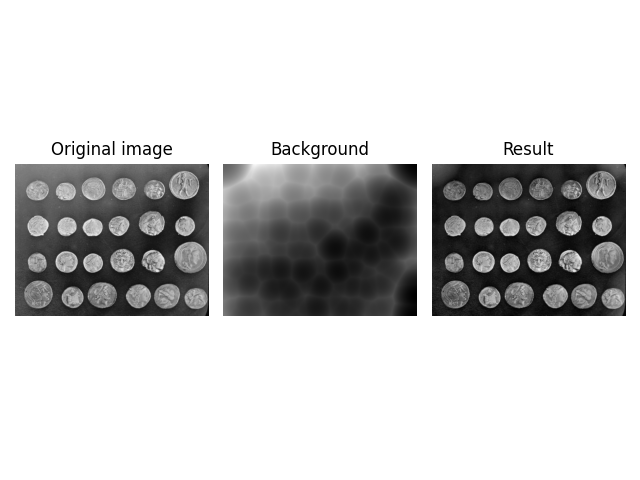By default, `rolling_ball` uses a ball shaped kernel (surprise). Sometimes, this can be too limiting - as in the example above -, because the intensity dimension has a different scale compared to the spatial dimensions, or because the image dimensions may have different meanings - one could be a stack counter in an image stack.

To account for this, `rolling_ball` has a `kernel` argument which allows you to specify the kernel to be used. A kernel must have the same dimensionality as the image (Note: dimensionality, not shape). To help with it’s creation, two default kernels are provided by `skimage`. `ball_kernel` specifies a ball shaped kernel and is used as the default kernel. `ellipsoid_kernel` specifies an ellipsoid shaped kernel.

```image = data.coins()
kernel = restoration.ellipsoid_kernel(
(70.5 * 2, 70.5 * 2),
70.5 * 2
)

background = restoration.rolling_ball(
image,
kernel=kernel
)
plot_result(image, background)
plt.show()
```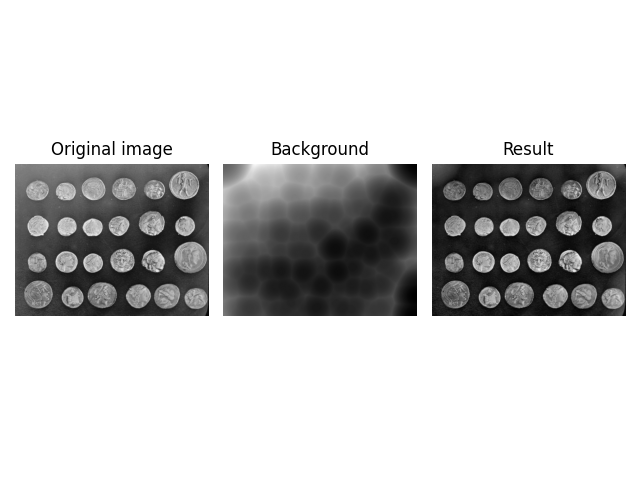You can also use `ellipsoid_kernel` to recreate the previous, unexpected result and see that the effective (spatial) filter size was reduced.

```image = data.coins()

kernel = restoration.ellipsoid_kernel(
(10 * 2, 10 * 2),
255 * 2
)

background = restoration.rolling_ball(
image,
kernel=kernel
)
plot_result(image, background)
plt.show()
```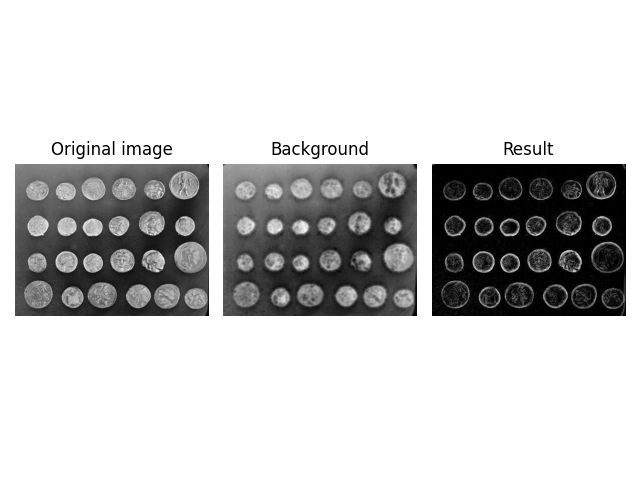## Higher Dimensions#

Another feature of `rolling_ball` is that you can directly apply it to higher dimensional images, e.g., a z-stack of images obtained during confocal microscopy. The number of kernel dimensions must match the image dimensions, hence the kernel shape is now 3 dimensional.

```image = data.cells3d()[:, 1, ...]
background = restoration.rolling_ball(
image,
kernel=restoration.ellipsoid_kernel(
(1, 21, 21),
0.1
)
)

plot_result(image[30, ...], background[30, ...])
plt.show()
```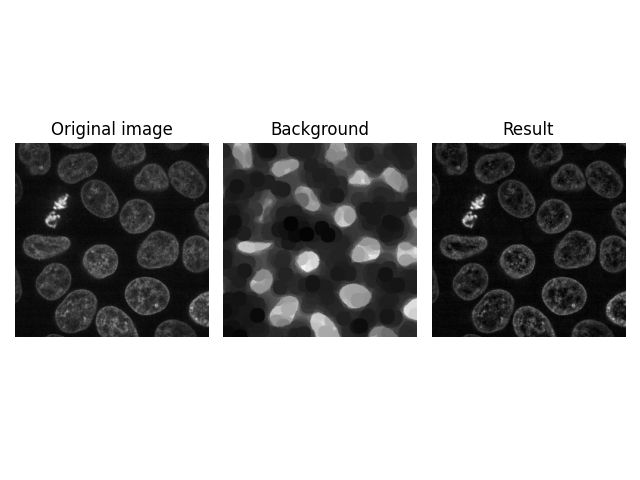A kernel size of 1 does not filter along this axis. In other words, above filter is applied to each image in the stack individually.

However, you can also filter along all 3 dimensions at the same time by specifying a value other than 1.

```image = data.cells3d()[:, 1, ...]
background = restoration.rolling_ball(
image,
kernel=restoration.ellipsoid_kernel(
(5, 21, 21),
0.1
)
)

plot_result(image[30, ...], background[30, ...])
plt.show()
```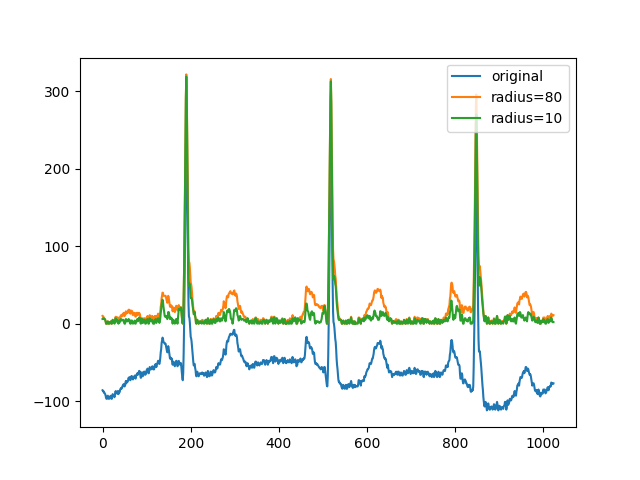Another possibility is to filter individual pixels along the planar axis (z-stack axis).

```image = data.cells3d()[:, 1, ...]
background = restoration.rolling_ball(
image,
kernel=restoration.ellipsoid_kernel(
(100, 1, 1),
0.1
)
)

plot_result(image[30, ...], background[30, ...])
plt.show()
```## 1D Signal Filtering#

As another example of the n-dimensional feature of `rolling_ball`, we show an implementation for 1D data. Here, we are interested in removing the background signal of an ECG waveform to detect prominent peaks (higher values than the local baseline). Smoother peaks can be removed with smaller values of the radius.

```x = pywt.data.ecg()